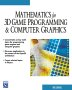# Maths - Kronecker Sum

### Notation

The symbolseems to be used to a number of related concepts:

• The Kronecker sum or tensor sum
• The direct sum
• Group direct product
• Direct product of modules

### The direct sum

A construction which combines several modules into a new, larger module. The result of the direct summation of modules is the "smallest general" module which contains the given modules as subspaces.

As far as I can tell, this does not presicely define the Cayley table, for example we could say:

H =³

Which says that quaternions are made up of a one dimensional scalar combined with a 3 dimensional vector like (actually bivector) quantity. Or:

H =CCj

Which says that quaternions are made up of two complex number algebras, or:

G3+ =²³

Which says that the even geometric algebra based on 3 dimensions which square to +ve is made up of a one dimensional scalar combined with a 3 dimensional bivector quantity.

### Group direct product

For abelian groups (groups which commute) we can represent the group operation asinstead of the usual multipication, in this case the identity element will be '0' and the inverse operation will be -x.

We can also use this to combine algebras, for instance if we have the algebras G and H with sample elements g and h. Combining these algebras can be defined elementwise:

(g, h) × (g' , h' ) = (g * g' , h o h' )

where:

• × is the operation of the combined algebra.
• * is the operation of the group G.
• o is the operation of the group H which may be, or maynot be, the same as *.

### Direct product of modules

The direct product for modules (not to be confused with the tensor product) is very similar to the one defined for groups above, using the cartesian product with the operation of addition being componentwise, and the scalar multiplication just distributing over all the components. Starting from R we get Euclidean space Rn, the prototypical example of a real n-dimensional vector space. The direct product of Rm and Rn is Rm + n.

If we are multiplying two integers n and m then we could say,

 n n*m = ∑ m 1=1

In the same way if we are raising n to the mth power then we could say,

 n nm = ∏ m 1=1

A direct product for a finite index is identical to the direct sum:

 n n= ∏ i=1 1=1

The direct sum and direct product differ only for infinite indices, where the elements of a direct sum are zero for all but for a finite number of entries. They are dual: the direct sum is the coproduct, while the direct product is the product.

## Cartesian and Tensor Products in other Algebras

Graphs Matrix
Cartesian Cartesian product Kronecker sum
Tensor tensor product Kronecker product

Cartesian and tensor products not only occur in matrix algebra but also occur in other algebras. It may help intuative understanding to look at these products in graph theory on the page here.

### Other meanings ofThe symbolis also used for the binary 'exclusive or' operation although

### Kronecker Sum of matrix with real terms

The Kronecker sum (or tensor sum) of A and B, denoted AB, is the mn×mn matrix:

AB = (ImA) + (BIn).

where:

• ARn×n
• BRm×m

### Example

In order to illustrate now to calculate the Kronecker sum here is an example:

If A =
 3 4 9 -4 3 6 3 -7 8
and B =
 5 -3 2 4

calculate AB

So in this case A is 3×3 so In=
 1 0 0 0 1 0 0 0 1
and B is 2 ×2 so Im=
 1 0 0 1

So AB = (ImA) + (BIn) gives:

AB=
 3 4 9 0 0 0 -4 3 6 0 0 0 3 -7 8 0 0 0 0 0 0 3 4 9 0 0 0 -4 3 6 0 0 0 3 -7 8
+
 5 0 0 -3 0 0 0 5 0 0 -3 0 0 0 5 0 0 -3 2 0 0 4 0 0 0 2 0 0 4 0 0 0 2 0 0 4

which gives

AB=
 8 4 9 -3 0 0 -4 8 6 0 -3 0 3 -7 13 0 0 -3 2 0 0 7 4 9 0 2 0 -4 7 6 0 0 2 3 -7 12

### General Case

Given K square matrices: AK,…,A1, where Al is of size nl×nl,

their Kronecker sum isK≥l≥1
Al=
 ∑ K≥l≥1

InK…nl+1AlInl−1…n1

∈ RnK…n1×nK…n1

where:

• Im = identity matrix of size m × m
• A[i,j] = AK[iK,jK] · AK−1[iK−1,jK−1]…A1[i1,j1]

using the mixed-base numbering scheme (indices start at 0)
i = (...((iK) · nK−1+ iK−1) · nK−2…) · n1+ i1= ∑K≥l≥1il· ∏l>h≥1nh

nonzeros:

η ( ⊕K≥l≥1Al) ≤∑K≥l≥1η(Al)nl· ∏K≥h≥1

### Properties

The Kronecker sum commutes:

AB = BA.

 metadata block see also: Correspondence about this page Book Shop - Further reading. Where I can, I have put links to Amazon for books that are relevant to the subject, click on the appropriate country flag to get more details of the book or to buy it from them.Mathematics for 3D game Programming - Includes introduction to Vectors, Matrices, Transforms and Trigonometry. (But no euler angles or quaternions). Also includes ray tracing and some linear & rotational physics also collision detection (but not collision response). Specific to this page here:

This site may have errors. Don't use for critical systems.